# Sparse Representation in a Gabor Dictionary¶

Important: Please read the installation page for details about how to install the toolboxes. $\newcommand{\dotp}{\langle #1, #2 \rangle}$ $\newcommand{\enscond}{\lbrace #1, #2 \rbrace}$ $\newcommand{\pd}{ \frac{ \partial #1}{\partial #2} }$ $\newcommand{\umin}{\underset{#1}{\min}\;}$ $\newcommand{\umax}{\underset{#1}{\max}\;}$ $\newcommand{\umin}{\underset{#1}{\min}\;}$ $\newcommand{\uargmin}{\underset{#1}{argmin}\;}$ $\newcommand{\norm}{\|#1\|}$ $\newcommand{\abs}{\left|#1\right|}$ $\newcommand{\choice}{ \left\{ \begin{array}{l} #1 \end{array} \right. }$ $\newcommand{\pa}{\left(#1\right)}$ $\newcommand{\diag}{{diag}\left( #1 \right)}$ $\newcommand{\qandq}{\quad\text{and}\quad}$ $\newcommand{\qwhereq}{\quad\text{where}\quad}$ $\newcommand{\qifq}{ \quad \text{if} \quad }$ $\newcommand{\qarrq}{ \quad \Longrightarrow \quad }$ $\newcommand{\ZZ}{\mathbb{Z}}$ $\newcommand{\CC}{\mathbb{C}}$ $\newcommand{\RR}{\mathbb{R}}$ $\newcommand{\EE}{\mathbb{E}}$ $\newcommand{\Zz}{\mathcal{Z}}$ $\newcommand{\Ww}{\mathcal{W}}$ $\newcommand{\Vv}{\mathcal{V}}$ $\newcommand{\Nn}{\mathcal{N}}$ $\newcommand{\NN}{\mathcal{N}}$ $\newcommand{\Hh}{\mathcal{H}}$ $\newcommand{\Bb}{\mathcal{B}}$ $\newcommand{\Ee}{\mathcal{E}}$ $\newcommand{\Cc}{\mathcal{C}}$ $\newcommand{\Gg}{\mathcal{G}}$ $\newcommand{\Ss}{\mathcal{S}}$ $\newcommand{\Pp}{\mathcal{P}}$ $\newcommand{\Ff}{\mathcal{F}}$ $\newcommand{\Xx}{\mathcal{X}}$ $\newcommand{\Mm}{\mathcal{M}}$ $\newcommand{\Ii}{\mathcal{I}}$ $\newcommand{\Dd}{\mathcal{D}}$ $\newcommand{\Ll}{\mathcal{L}}$ $\newcommand{\Tt}{\mathcal{T}}$ $\newcommand{\si}{\sigma}$ $\newcommand{\al}{\alpha}$ $\newcommand{\la}{\lambda}$ $\newcommand{\ga}{\gamma}$ $\newcommand{\Ga}{\Gamma}$ $\newcommand{\La}{\Lambda}$ $\newcommand{\si}{\sigma}$ $\newcommand{\Si}{\Sigma}$ $\newcommand{\be}{\beta}$ $\newcommand{\de}{\delta}$ $\newcommand{\De}{\Delta}$ $\newcommand{\phi}{\varphi}$ $\newcommand{\th}{\theta}$ $\newcommand{\om}{\omega}$ $\newcommand{\Om}{\Omega}$

This numerical tour explores the use of L1 optimization to find sparse representation in a redundant Gabor dictionary. It shows application to denoising and stereo separation.

In :
addpath('toolbox_signal')


## Gabor Tight Frame Transform¶

The Gabor transform is a collection of short time Fourier transforms (STFT) computed with several windows. The redundancy |K*L| of the transform depends on the number |L| of windows used and of the overlapping factor |K| of each STFT.

We decide to use a collection of windows with dyadic sizes.

Sizes of the windows.

In :
wlist = 32*[4 8 16 32];
L = length(wlist);


Overlap of the window, so that |K=2|.

In :
K = 2;
qlist = wlist/K;


Overall redundancy.

In :
disp( strcat(['Approximate redundancy of the dictionary=' num2str(K*L) '.']) );

Approximate redundancy of the dictionary=8.


In :
n = 1024*32;
options.n = n;


Compute its short time Fourier transform with a collection of windows.

In :
options.multichannel = 0;
S = perform_stft(x0,wlist,qlist, options);


Exercise 1

Compute the true redundancy of the transform. Check that the transform is a tight frame (energy conservation).

In :
exo1()

True redundancy of the dictionary=8.0586.

In :
%% Insert your code here.


Display the coefficients.

In :
plot_spectrogram(S, x0);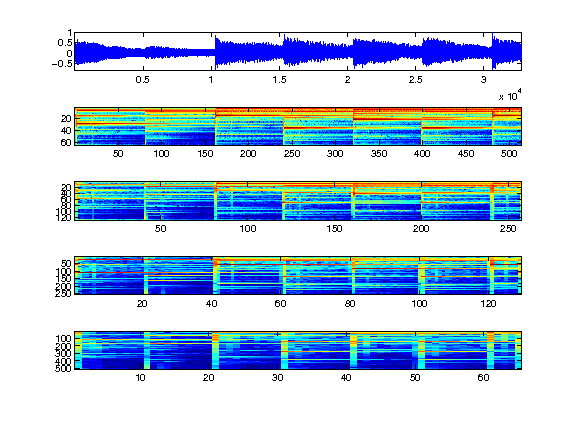Reconstructs the signal using the inverse Gabor transform.

In :
x1 = perform_stft(S,wlist,qlist, options);


Check for reconstruction error.

In :
e = norm(x0-x1)/norm(x0);
disp(strcat(['Reconstruction error (should be 0) = ' num2str(e, 3)]));

Reconstruction error (should be 0) = 1.58e-16


## Gabor Tight Frame Denoising¶

We can perform denoising by thresholding the Gabor representation.

We add noise to the signal.

In :
sigma = .05;
x = x0 + sigma*randn(size(x0));


Denoising with soft thresholding. Setting correctly the threshold is quite difficult because of the redundancy of the representation.

transform

In :
S = perform_stft(x,wlist,qlist, options);


threshold

In :
T = sigma;
ST = perform_thresholding(S, T, 'soft');


reconstruct

In :
xT = perform_stft(ST,wlist,qlist, options);


Display the result.

In :
err = snr(x0,xT);
clf
plot_spectrogram(ST, xT);
subplot(length(ST)+1,1,1);
title(strcat(['Denoised, SNR=' num2str(err,3), 'dB']));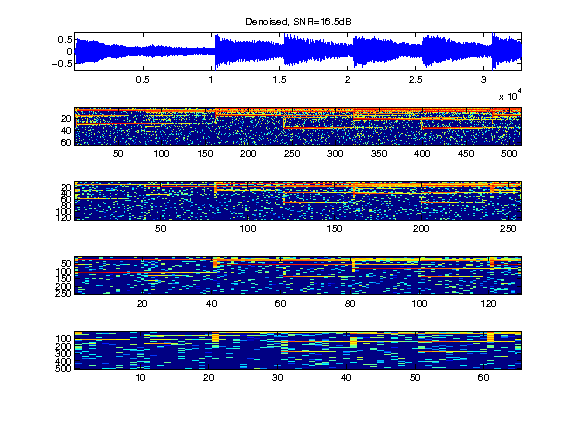Exercise 2

Find the best threshold, that gives the smallest error.

In :
exo2()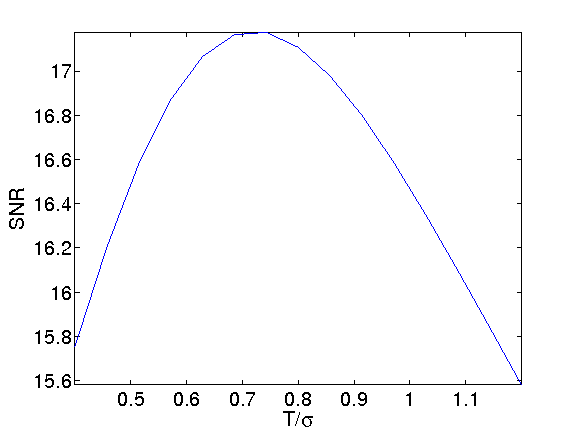In :
%% Insert your code here.


## Basis Pursuit in the Gabor Frame¶

Since the representation is highly redundant, it is possible to improve the quality of the representation using a basis pursuit denoising that optimize the L1 norm of the coefficients.

The basis pursuit finds a set of coefficients |S1| by minimizing

|min_{S1} 1/2norm(x-x1)^2 + lambdanorm(S1,1) (*)|

Where |x1| is the signal reconstructed from the Gabor coefficients |S1|.

The parameter |lambda| should be optimized to match the noise level. Increasing |lambda| increases the sparsity of the solution, but then the approximation |x1| deviates from the noisy observations |x1|.

Basis pursuit denoising |(*)| is solved by iterative thresholding, which iterates between a step of gradient descent, and a step of thresholding.

Initialization of |x1| and |S1|.

In :
lambda = .1;
x1 = x;
S1 = perform_stft(x1,wlist,qlist, options);


Step 1: gradient descent of |norm(x-x1)^2|.

residual

In :
r = x - x1;
Sr = perform_stft(r, wlist, qlist, options);


Step 2: thresholding and update of |x1|.

threshold

In :
S1 = perform_thresholding(S1, lambda, 'soft');


update the denoised signal

In :
x1 = perform_stft(S1,wlist,qlist, options);


The difficulty is to set the value of |lambda|. If the basis were orthogonal, it should be set to approximately 3/2*sigma (soft thresholding). Because of the redundancy of the representation in Gabor frame, it should be set to a slightly larger value.

Exercise 3

Perform the iterative thresholding by progressively decaying the value of |lambda| during the iterations, starting from |lambda=1.5sigma| until |lambda=.5sigma|. Retain the solution |xbp| together with the coefficients |Sbp| that provides the smallest error.

In :
exo3()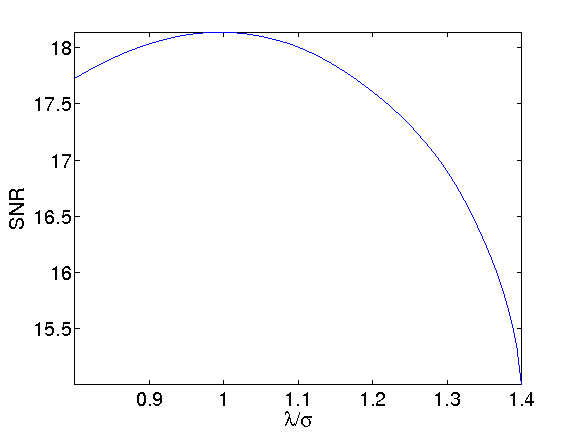In :
%% Insert your code here.


Display the solution computed by basis pursuit.

In :
e = snr(x0,xbp);
clf
plot_spectrogram(Sbp, xbp);
subplot(length(Sbp)+1,1,1);
title(strcat(['Denoised, SNR=' num2str(e,3), 'dB']));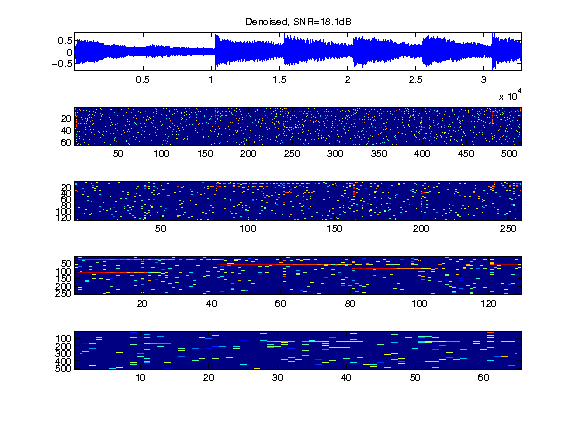## Sparsity to Improve Audio Separation¶

The increase of sparsity produced by L1 minimization is helpful to improve audio stereo separation.

In :
n = 1024*32;
options.n = n;
s = 3; % number of sound
p = 2; % number of micros
options.subsampling = 1;
x = zeros(n,3);


normalize the energy of the signals

In :
x = x./repmat(std(x,1), [n 1]);


We mix the sound using a |2x3| transformation matrix. Here the direction are well-spaced, but you can try with more complicated mixing matrices.

compute the mixing matrix

In :
theta = linspace(0,pi(),s+1); theta(s+1) = [];
theta(1) = .2;
M = [cos(theta); sin(theta)];


compute the mixed sources

In :
y = x*M';


We transform the stero pair using the multi-channel STFT (each channel is transformed independantly.

In :
options.multichannel = 1;
S = perform_stft(y, wlist, qlist, options);


check for reconstruction

In :
y1 = perform_stft(S, wlist, qlist, options);
disp(strcat(['Reconstruction error (should be 0)=' num2str(norm(y-y1,'fro')/norm(y, 'fro')) '.' ]));

Reconstruction error (should be 0)=1.5966e-16.


Now we perform a multi-channel basis pursuit to find a sparse approximation of the coefficients.

regularization parameter

In :
lambdaV = .2;


initialization

In :
y1 = y;
S1 = S;
niter = 100;
err = [];


iterations

In :
for i=1:niter
r = y - y1;
Sr = perform_stft(r, wlist, qlist, options);
% multi-channel thresholding
%%% BUG HERE %%%%
% S1 = perform_thresholding(S1, lambdaV, 'soft-multichannel');
% update the value of lambdaV to match noise
y1 = perform_stft(S1,wlist,qlist, options);
end


Create the point cloud of both the tight frame and the sparse BP coefficients.

In :
P1 = []; P = [];
for i=1:length(S)
Si = reshape( S1{i}, [size(S1{i},1)*size(S1{i},2) 2] );
P1 = cat(1, P1,  Si);
Si = reshape( S{i}, [size(S{i},1)*size(S{i},2) 2] );
P = cat(1, P,  Si);
end
P = [real(P);imag(P)];
P1 = [real(P1);imag(P1)];


Display the two point clouds.

In :
p = size(P,1);
m = 10000;
sel = randperm(p); sel = sel(1:m);
clf;
subplot(1,2,1);
plot( P(sel,1),P(sel,2), '.' );
title('Tight frame coefficients');
axis([-10 10 -10 10]);
subplot(1,2,2);
plot( P1(sel,1),P1(sel,2), '.' );
title('Basis Pursuit coefficients');
axis([-10 10 -10 10]);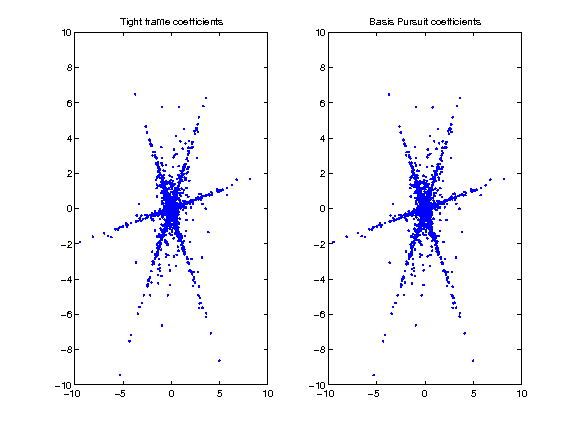Compute the angles of the points with largest energy.

In :
d  = sqrt(sum(P.^2,2));
d1 = sqrt(sum(P1.^2,2));
I = find( d>.2 );
I1 = find( d1>.2 );


compute angles

In :
Theta  = mod(atan2(P(I,2),P(I,1)), pi());
Theta1 = mod(atan2(P1(I1,2),P1(I1,1)), pi());


Compute and display the histogram of angles. We reaint only a small sub-set of most active coefficients.

compute histograms

In :
nbins = 150;
[h,t] = hist(Theta, nbins);
h = h/sum(h);
[h1,t1] = hist(Theta1, nbins);
h1 = h1/sum(h1);


display histograms

In :
clf;
subplot(2,1,1);
bar(t,h); axis('tight');
set_graphic_sizes([], 20);
title('Tight frame coefficients');
subplot(2,1,2);
bar(t1,h1); axis('tight');
set_graphic_sizes([], 20);
title('Sparse coefficients');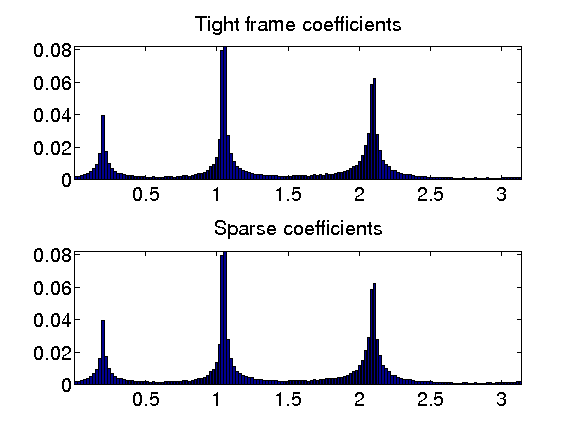Exercise 4

Compare the source separation obtained by masking with a tight frame Gabor transform and with the coefficients computed by a basis pursuit sparsification process.

In :
exo4()

In :
%% Insert your code here.

In [ ]: